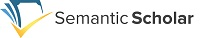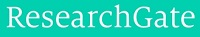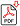Search for Articles:

 Indexing RepositoriesSponsors## A Bayesian Approach for Estimating the Scale Parameter of Double Exponential Distribution under Symmetric and Asymmetric Loss Functions

Md. Rashidul Hasan

Abstract: The main objective of this paper is to study the Bayes estimators of the parameter of Double Exponential distribution under different loss functions and then compared among them as well as with the classical estimator named maximum likelihood estimator (MLE). In our real life, we always try to minimize the loss and we also want to gather some prior information (distribution) about the problem to solve it accurately. Here the conjugate (Gamma) prior is used as the prior distribution of Double Exponential distribution for finding the Bayes estimator. In our study, we used different symmetric (squared error and quadratic) and asymmetric (MLINEX and NLINEX) loss functions. Finally, mean square error (MSE) of the estimators are obtained and then presented graphically.

Keywords: Bayes estimator, Maximum Likelihood Estimator (MLE), Squared Error (SE) Loss Function, Modified Linear Exponential (MLINEX) Loss Function, Non-Linear Exponential (NLINEX) Loss Function.

Pages: 351 - 356

Edition: Volume 8 Issue 3, March 2019